Chapter Notes: Polynomials

# Polynomials Chapter Notes - Mathematics (Maths) Class 9

## Introduction to Polynomial

The word Polynomial comes from poly - means "many" and - nomial means "term". It means that it contains "many terms". Polynomials are subset of algebraic expressions. Polynomials are algebraic expressions that may contain exponents which are added, subtracted or multiplied.

“The expression which contains one or more terms with non – zero coefficient is called polynomial”

An algebraic form of the Polynomial is:
p(X) = anxn +an-1Xn-1 + an-2Xn-2 +... a2X2 + a1X +a0
Where, n is a positive integer and a0, a1, a2, a3, a4, ... ... ... . . , a: are constants and a ≠ 0. They are also known as coefficients of polynomials.
Now, let us discuss an example of polynomial and what it contains.
The example of a polynomial is: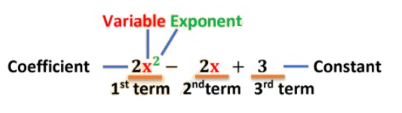So, a Polynomial can have constants, variables, exponents, terms and coefficient.

(i) Constant: A symbol having a fixed numerical value is called a constant.
Example: 1, 2, π = 3.14, √2 = 1.414 etc.... are the examples of constants because their value is fixed and do not change.

Let us consider an example here,
Find the circumference of the two circles having radius 2 cm and 4 cm respectively.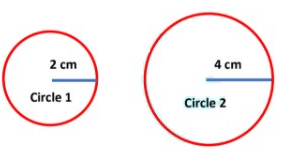r(Radius of small circle) = 2 cm
r(Radius of big circle) = 4 cm
We know that,
Circumference of the circle = 2πr
Circumference of the small circle = 2π × r1 = 2π × 2 = 4π ......... (1)
Circumference of the second circle = 2π × r2 = 2π × 4 = 8π .....(2)
From equations 1 and 2, we see that the value of 2π is constant and it does not change anywhere.

(ii) Variable: A symbol which may be assigned different values is called a variable.
Example: Letters x, y, z, a, b, c, r, etc.... they are used to denote variable that can take any real value.

Again consider the example of the two circles.
Find the circumference of the two circles having radius 2 cm and 4 cm respectively.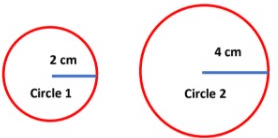r(Radius of small circle) = 2 cm
r(Radius of big circle) = 4 cm
We know that,
Circumference of the circle = 2πr
Circumference of the small circle = 2π × r1 = 2π × 2 = 4π .........(1)
Circumference of the big circle = 2π × r2 = 2π × 4 = 8π .....(2)
From equations 1 and 2 we see that the values of variables r1 and r2 vary in both the cases.

(iii) Terms: Various parts of an expression which are separated by the sign of addition or subtraction are called the terms of an algebraic expression.
Example: 2x2  -  2x + 3

In the given polynomial, expressions 2x2, 2x and 3 are called the terms of the polynomial. These are separated by subtraction and addition signs.

There are two types of terms present in the Polynomial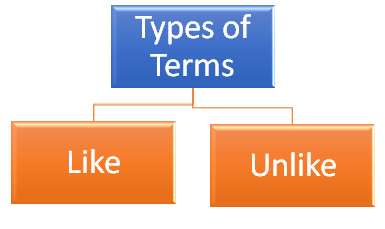(a) Like terms: Like terms are terms that have same variables and powers. The coefficients do not need to match.
Example:(b) Unlike terms: Unlike terms are two or more terms that are not like terms, i.e. they do not have the same variables or powers.
Example: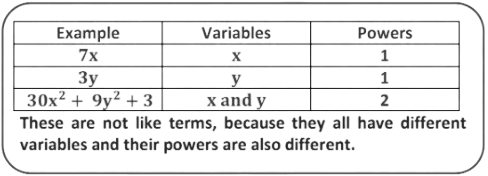(iv) Coefficient: The numerical factor is said to be the numerical coefficient or simply the coefficient of the term. Each term of a polynomial has coefficient.
Example:

2x2  -  2x + 3
= 2x2 − 2x + 3x0 ....... (x0 = 1 )
The coefficient of x2 is 2,
Coefficient of x is − 2
Coefficient of x° is 3

(v) Exponent: The exponent of a number tells how many times to use that number in a multiplication. In polynomials, an exponent is always a whole number ( 0, 1, 2, 3, ... . ).
Example: 2x2 -  2x + 3

In the given example, exponent of x in the first term is 2

Consider the examples of
(i)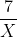and (ii) 3 √Z.

(i)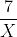= 7x-1. Here, the exponent of x is −1, which is not a whole number. Therefore, this expression is not a polynomial.
(ii) 3 √Z = 3z1/2. Here, the exponent of z is 1/2 , which is not a whole number. Therefore, this expression is not a polynomial.
Now, we can just recall what is polynomial? And what it contains.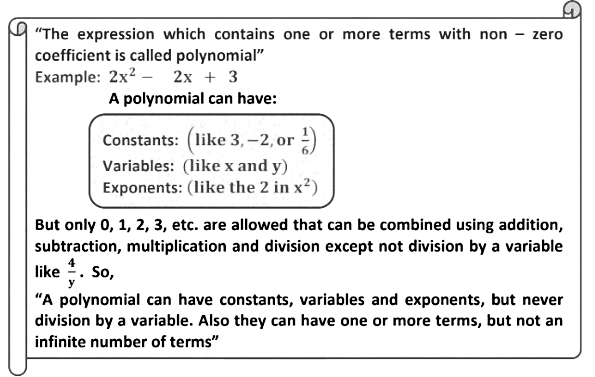Which of the following are the examples of polynomial and which are not?
(i) 2x
(ii) y – 2
(iii) 6
(iv) 5a-1b-3
(v) 2x2 + 5x + 50
(vi) √7
(vii)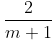(viii) √n
(ix)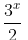(i) 2x  = 2x1

Is a polynomial, because it contain only one variable x and the exponent of x is 1, which is a whole number.
(ii) y - 2
Is a polynomial, because it contains only one variable y and the exponent of y is 1 and the exponent of 2 is 0, which is a whole number
(iii) 6
6 = 6x0 is the polynomial because it contains only one variable x and the exponent of x is 0, which is a whole number
(iv) 5a-1b-3
Is not a polynomial, because it contains two variables a and b and the exponent of a and b is −1 and − 3, which is not a whole number.
(v) 2x2 + 5x + 50
Is a polynomial, because in this expression the exponent of x2 is 2, x is 1 and the exponent of 50 is 0 which is the whole number.
(vi) √7
Is a polynomial, because the value of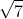is constant
(vii)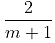Is not a polynomial, because in polynomial division by a variable is not allowed
(viii) √n = n x 1/2
Is not a polynomial, because the exponent of n is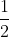, which is not a  whole number.
(ix)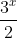=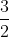x1
Is a polynomial, because you can divide an expression by a constant and the exponent of x is 1, which is a whole number.

Example: Write the coefficients of x2 in each of the following

(i) 17– 2x + 7x2
(ii) 9– 12x + x2
(iii) πx2 − 2x + 1
We know that a numerical factor is the coefficient of the term.
(i) 17 - 2x + 7x2

The coefficient of xin this expression is 7.

(ii) 9 - 12x + x2

The coefficient of x2 in this expression is 1.

(iii) πx2 - 2x + 1

The coefficient of x2 in this expression is π
Find the coefficients of x2 in (2x + x2) (x + 1/x )
We have, (2x + x2) (x +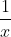)
=2x X x + 2x  X+ x2 X x + x2 X=2x2 + 2 +x3 +x = x3 + 2x2 + x + 2
So, the coefficient of x2 is 2

Question for Chapter Notes: Polynomials
Try yourself:Which of the following is NOT a polynomial?

### Classification of Polynomials

(1) Classification of polynomials on the basis of number of terms

On the basis of number of terms, the polynomials can be classified as: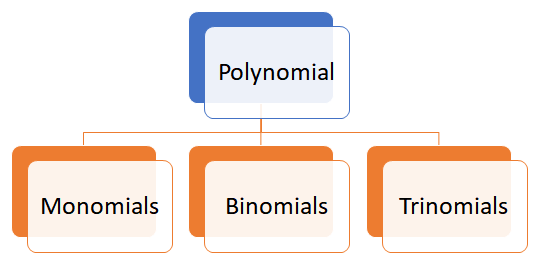(i) Monomial: An algebraic expression that contains only one term is called a monomial.

Example: 2x, 3, 4t, 9pq, 2c+ etc...

Each of these expressions contains only one term and hence is called monomial.

(ii) Binomial: An algebraic expression that contains only two unlike terms is called a binomial.

Example: (i) 6x + 2x2

The given expression is a binomial since it contains two unlike terms, that is, 6x and 2x+ which are separated by a plus sign.

(ii) 6x - 2x2

The given expression is a binomial since it contains two unlike terms, that is, 6x and 2x+ which are separated by a minus sign.
If the terms, 6x and 2x2 are separated by a multiplication sign (6x × 2x2) then it is not binomial because when we multiply two unlike terms then a monomial is formed. 6x × 2x2 = (6 × 2)(x × x2) = 12x3

(iii) Trinomial: An algebraic expression that contains only three unlike terms is called a trinomial.
Example: (i) x + x2 – 3x3

Is trinomial, since it contains three unlike terms namely, x, xand−3x3.

(ii) 7y3 + 8y2 + 9y

Is trinomial, since it contains three unlike terms namely, 9y, 8y2 and 7y3.

State which of the following is monomial, binomial and trinomial?
(i) 5 × a + a
(ii) 7a2 + 8b + 9c
(iii) 6a2 + 5b2
(iv) 3a × 4b + 2c
(i) 5 × a + a

= 5a + a = 6a
Here, number of terms is 1. Hence, it is a monomial.

(ii) 7a2 + 8b + 9c

Here, number of terms is 3. Hence, it is a trinomial.

(iii) 6a2 + 5b2

Here, number of terms is 2. Hence, it is a binomial.

(iv) 3a × 4b + 2c

= 12ab + 2c
Here, number of terms is 2. Hence, it is a binomial.

(2) Classification of polynomials on the basis of degree of variables
On the basis of degree of variable, polynomials are classified as: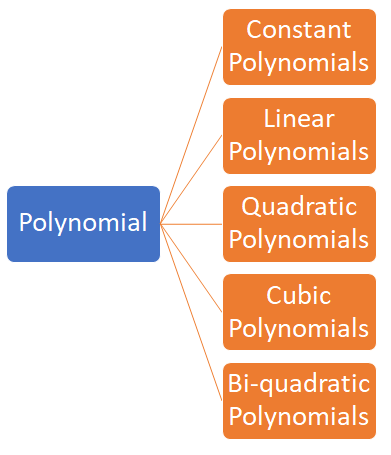(i) Constant polynomial: A polynomial of degree zero is called a constant polynomial.
Example: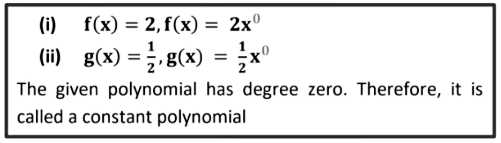(ii) Linear polynomial: A polynomial of degree one is called a linear polynomial. Linear polynomial may be a monomial or a binomial.
Example: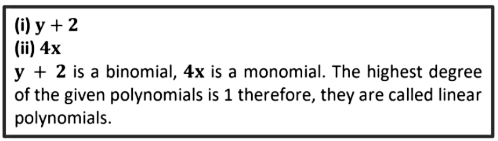(iii) Quadratic polynomial: A polynomial of degree two is a called quadratic polynomial. Quadratic polynomial may be a monomial or a binomial or a trinomial.
Example: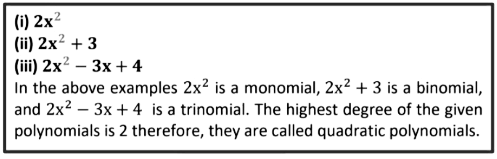(iv) Cubic polynomial: A polynomial of degree three is called cubic polynomial.
Example: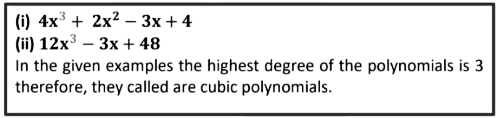(v) Biquadratic polynomial: A polynomial of degree four is called a biquadratic polynomial.
Example: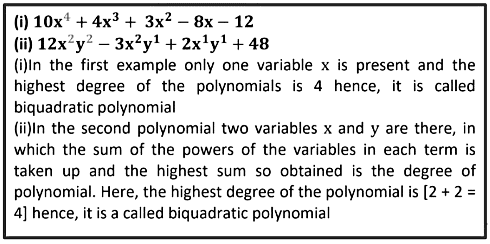Identify the type of the polynomials given below (on the basis of degree)
(i) 5y + 3
(ii) √3+ x2 + x
(iii) y+ y2 + y + 1
(i) 5y + 3

In this polynomial, the highest power of y is 1, so it is a linear polynomial

(ii) √3 + x+ x

In this polynomial, the highest power of x is 2, so it is a quadratic polynomial

(iii) y3+ y2 + y + 1

In this polynomial, the highest power of y is 3, so it is a cubic polynomial.

Zero polynomial: If a0 = a1 = a2 = ⋯ a= 0 (all constants are zero), then we get the zero polynomial. If p(n) = 0, then it is called a zero polynomial. The degree of zero polynomial is not defined. It is denoted by 0.

### Polynomial in One Variable

“An expression which consists of only one type of variable in the entire expression is called polynomial in one variable”.

OR

“An algebraic expression involving single variable, which have only whole number as the exponent of the variable are called polynomial in one variable”.
If the variable in the polynomial is x then we denote the polynomial by p(x) or q(x), etc....
A polynomial in one variable x , is an algebraic expression of the form
p(X) = anxn +an-1Xn-1 + an-2Xn-2 +... a2X2 + a1X +a0
Where, n is a positive integer and a0, a1, a2, a3, a4, ... ... ... . . , an are constants and a: ≠ 0. They are also known as coefficients of polynomials.
Example:
(i) 5x3 + 2x2 − 7x + 1 is a polynomial in one variable x
(ii) 3y2 + 7y is a polynomial in one variable y
Now, let’s solve some examples:
Which of the following expressions are polynomials in one variable and which are not?

(i) 2x2– x + 8
(ii) y +1/6
(iii) x15 + y2 + t25
(i) 2x2– x + 8

In this expression only one variable x is used. Hence, it is called polynomial in one variable.

(ii) y + 1/6

In this expression only one variable y is used. Hence, it is called polynomial in one variable.

(iii) x15 + y2 + t25

In this expression three variables x, y and t are used. Hence, it is called polynomial in three variables.

### Degree of a polynomial

“Highest power of variable in a polynomial is called degree of polynomial.”

Example:
(i) 2t4 − 3t3 + 2t + 1 is a polynomial in t of degree 4. Since the highest power of t is 4.
(ii) 3/5 + 1/5x + 5x2 + 3/2 x3 + x5 is a polynomial in x of degree 5. Since, the highest power of x is 5.

(1) Degree of polynomial in one variable: In the polynomial in one variable, the highest power of the variable is called the degree of polynomial in one variable.
Example:
(i) √2 + 4x is a polynomial in x of degree 1. Since the highest power of x is 1.
(ii) t5 + t3 + t + 1 is a polynomial in t of degree 5. Since the highest power of t is 5.

(2) Degree of polynomial in two or more variables:
The sum of the powers of the variables in each term is taken up and the highest sum so obtained is called the degree of a polynomial in two or more variables.
Example:
(i) x1y5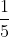x2y3+x1y1-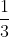Is the polynomial in two variable x and y, in which the sum of the powers of the variables in each term is taken up and the highest sum so obtained is the degree of polynomial i.e., [1 + 5 = 6.]

(ii) 4a2b4c1 − 3a1b2c2 + 2a1b1c1 − 7

Is the polynomial in three variable a, b and c. In which the sum of the powers of the variables in each term is taken up and the highest sum so obtained is the degree of polynomial i.e., [2 + 4 + 1 = 7].

Write the degree of the following polynomials.
(i)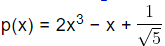(ii)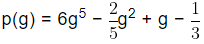(iii) p(x) = 5x2 + 2x + 5
(i)Since, the highest power of x in p(x) is 3, the degree of polynomial 2x3− x +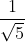is 3.

(ii)As the highest power of g in the polynomial, p(g) is 5, hence the degree of polynomial 6g5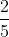g2 + g −is 5.

(iii) p(x) = 5x2 + 2x + 5

As the highest power of x in p(x) is 2, hence the degree of polynomial p(x) = 5x2 + 2x + 5 is 2

Question for Chapter Notes: Polynomials
Try yourself:The degree of a polynomial is:

### Zeroes of a Polynomial

Value of polynomial:
The value of polynomial obtained by putting a particular value of the variable is called value of polynomial.
The value of a polynomial p(x) at x = a is denoted by p(a)
Example:

p(x) = 4x3 − 2x3 + 2x − 2
At x = 1
p(1) = 4(1)3 − 2(1)3 + 2(1) − 2
p(1) = 4 − 2 + 2 − 2
p(1) = 2 + 2 − 2
p(1) = 4 − 2 = 2
So, 2 is the value of given polynomial p(x) at x = 1

Find the value of each of the following polynomials at the indicated value of variables.
(i) q(y) = 2y3 − 2y + √10 at y = 2
(ii) p(r) = 4r2 − 2r + 6 at r = a

(i) q(y) = 2y3 − 2y + √10 at y = 2

On putting y = 2 in q(y) we get,
q(2) = 2(2)3 − 2(2) + √10
q(2) = 2 × 8 − 4 + √10
q(2) = 16 − 4 + √10
q(2) = 12 + √10 , which is the required value of q(y) at 2

(ii) p(r) = 4r2 − 2r + 6 at r = a

On putting r = a in p(r) we get,
p(a) = 4(a)2 − 2(a) + 6
p(a) = 4a2 − 2a + 6, which is the required value of p(r) at r = a

Zero of a polynomial: Zero of a polynomial p(x) is a number a, such that p(a) = 0. Zero

of a polynomial is also called root of the polynomial, p(x) = 0.
Example:

p(x) = x – 3, at x = 3
p(3) = 3 − 3 = 0.
Thus 3 is a zero of polynomial p(x) = x − 2
Zero of a polynomial is special. It is used to find factors of the polynomial.

Example: Find the zero of polynomial 3x+ 6

Given polynomial p(x) = 3x + 6
On putting p(x) = 0, we get 3x + 6 = 0
3x = 0 − 6
3x = −6
x =  6/3  =  -2
Hence, x = −2 i.e., p(−2) = 0 is the zero of polynomial, 3x + 6

Check whether −2 and 2 are zeros of the polynomial t2− t − 6

The given polynomial is t7 − t − 6........ (1)
On putting t = −2 in equation (1) we get,
p(t) = t2 − t − 6
p(−2) = (−2)2 − (−2) − 6
p(−2) = 4 + 2 − 6
p(−2) = 6 − 6
p(−2) = 0
Again, on putting t = 2 in equation (1) we get,
p(t) = t2 − t − 6
p(2) = (2)2 − (2) − 6
p(2) = 4 − 2 − 6
p(2) = 2 − 6
p(2) = −4
Therefore, –2 is a zero of the polynomial t2 − t − 6, but 2 is not as the value of p(2) is not equal to zero.

If x = 2 is a root of the polynomial, f(x) = 2x2– 3x + 6a, Find the value of a.

We know that, f(x) = 2x2– 3x + 6a .... (1)
Given: x = 2 is the root of f(x)
On putting x = 2 in equation (1) we get,
f(2) = 2(2)2– 3(2) + 6a
f(2) = 2 × 4 – 3(2) + 6a
f(2) = 8 – 6 + 6a
f(2) = 6a + 2
Now, equate 6a + 2 = 0
6a + 2 = 0
6a = −2
a = 2/6  =  1/3
The value of a =1/3

### Important points on zeroes of a polynomial

(1) Zero may be the zero of a polynomial.

Example:
(i) p(x) = x2

x = 0
P(0) = 0

(ii) p(x) = x + 5

x = 0
p(0) = 0 + 5
p(0) = 5
From the above two examples, we see that zero may or may not be the zero of a polynomial.

(2) Every linear polynomial has a unique zero.
Example:
Consider the linear polynomial p(x) = 3x + 6

For p(x) = 0
3x + 6 = 0
3x = −6
x = −6/3
x = −2
Therefore for x = −2, the value of a polynomial is 0 and hence, x = −2 is a zero of a given polynomial. There is no other value is possible other than x = −2 for which p(x) = 0 that’s how every linear polynomial has unique zero.

(3) Zero of a polynomial is called root of the polynomial.

p(x) = 0
p(x) = ax + b
∴ ax + b = 0
ax = −b
x = −b/a
x = −b/a it is the zero of polynomial.
It is also called the root of the polynomial p(x) = ax + b

(4) Every non-zero constant polynomial has no zero.
Example:
Consider the constant polynomial

p(x) = 3 i.e.,
It can be written as p(x) = 3x0......(i)
When we place any number in 3x0still, we get 3
[Because for any value x0 = 1]

Example:

(1) Let put x0 = 2 in equation (i) then
p(x) = 3x0= 3 × 20 = 3
(2) Let put x0 = 100 in equation (i) then
p(x) = 3x0 = 3 × 1000 = 3
From the above two example we see that when we place any number in 3x0 still, we get 3.

(5) Every real number is a zero of the zero polynomial

Example:

p(x) = ax2 + bx
In zero polynomial, all the coefficients are zero hence, a = b = 0
p(x) = 0. x2 + 0. x = 0
For every real number value of x p(x) is zero in this case that’s how every real number is a zero of zero polynomial.

• Example 1: p(x) = 0. x2 + 0. x
Put x = 1
p(1) = 0. (1)2 + 0. (1) = 0
• Example 2: p(x) = 0. x2 + 0. x
Put x = −1
p(−1) = 0. (−1)2 + 0. (−1) = 0

From the above two example we see that every real number is a zero of the zero polynomial

(6) A polynomial can have more than one zero

Example: 4x4 + 0. x3 − 0. x2 + 3x + 6. This polynomial consist of more than one zero.

Consider a polynomial p(x) = x2 − 1
Let x = 1
For p(x) = 0
p(x) = x2 − 1
p(1) = (1)− 1
p(1) = 1 − 1
p(1) = 0
Let x = −1
For p(x) = 0
p(x) = x2 − 1
p(−1) = (−1)2 − 1
p(−1) = 1 − 1
p(−1) = 0
From the above example we see that a polynomial can have more than one zero

### Factorisation of Polynomials

Factor theorem

Let p(x) be a polynomial of degree n ≥ 1 and a be any real number such that,

(i) p(a) = 0, then (x − a) is a factor of p(x) conversely,
(ii)If (x − a) is a factor of p(x), then p(a) = 0
Proof:

(i) Given p(a) = 0
Now, suppose p(x) is divided by (x − a), then quotient is g(x).
By remainder theorem, when p(x) is divided by (x − a), then remainder is p(a).
Dividend = Divisor × Quotient + Remainder
∴ p(x) = (x − a). g(x) + p(a)
p(x) = (x − a). g(x) + 0 [∵ p(a) = 0]
So, (x − a) is a factor of p(x)
(ii) Let (x − a) be a factor of p(x)
On dividing p(x) by (x − a), let g(x) be the quotient.
∴ p(x) = (x − a). g(x)
On putting x = a, we get
p(a) = (a − a). g(a)
p(a) = 0. g(a)
p(a) = 0
Thus, if (x − a) is a factor of p(x), then p(a) = 0

Examine whether x + 1 is a factor of p (x) = x3 + 3x3 + 5x + 6
Let p(x) = x+ 3x2 + 5x + 6
By factor theorem, (x − a) is a factor of p(x) if p(a) = 0.
Therefore, in order to check that (x + 1) is a factor of p(x), it is sufficient to check that if p(−1) = 0 then it is a factor otherwise not.
p(x) = x3 + 3x2 + 5x + 6
p(– 1) = (– 1)3+ 3(– 1)2 + 5(– 1) + 6
p(– 1) = −1 + 3 − 5 + 6
p(– 1) = −6 + 9
p(– 1) = 3 ≠ 0
Thus, x + 1 is NOT a factor of p(x) = x3 + 3x2 + 5x + 6

Find the value of a if x-a is a factor of x3 - a2x + x + 2
Let p(x) = x3 − a2x + x + 2
By factor theorem, (x − a) is a factor of p(x) if p(a) = 0
Now, p(a) = a3 − a2 × a + a + 2
a− a2 × a + a + 2 = 0
a3 − a3 + a + 2 = 0
a + 2 = 0
a = 0 − 2
a = −2
Hence, (x − a) is a factor of the given polynomial, if a = −2

Quadratic polynomial is represented as ax2 + bx + c, where a, b, c are constants and a ≠ 0 . It can be factorised by different methods.
(i) By splitting the middle term
(ii) By using factor theorem
(i) By splitting middle term
Let the factor of quadratic polynomial ax2 + bx + c be (px + q) and (rx + s). Then
ax+ bx + c = (px + q)(rx + s)
= prx2 + (ps + qr)x + qs
Now,
Comparing x2, x and constant terms, we get
a = pr, b = ps + qr and c = qs
Here, b is the sum of two numbers ps and qr, whose product is (ps)(qr) = (pr)(qs) = ac
Thus, to factories ax2 + bx + c , write b as the sum of two numbers, whose product is ac.

Factorizing 2x2 + 7x + 3 by splitting the middle term
Given polynomial is 2x2 + 7x + 3 .
On comparing with ax2 + bx + c, we get
a = 2, b = 7 and c = 3
Now ac = 2 × 3 = 6
So, all possible pairs of factors 6 is 1 and 6, 2 and 3.
Pair 1 and 6 give 1 + 6 = 7 = b
∴ 2x2 + 7x + 3 = 2x2 + (1 + 6)x + 3
= 2x2 + x + 6x + 3
= x(2x + 1) + 3(2x + 1)
= (2x + 1)(x + 3)

(ii) By using factor theorem
Write the given polynomial p(x) = ax2 + bx + c in the form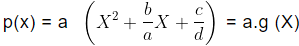Where,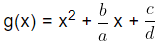i.e. firstly make the coefficient of x2 equal to 1 if it is not 1.

Factorise x2 - 5x +  6 by using factor theorem.
Let given polynomial be f(x) = x2 − 5x + 6
Here, the coefficient of x2 is 1, so we do not need to write it in the form of a. g(x).
Now, constant term is 6 and all factors of 6 are ±1, ±2, ±3 and ± 6
At x = 2,
f(2) = (2)2 − 5(2) + 6
f(2) = 4 − 10 + 6
f(2) = −6 + 6
f(2) = 0
At x = 3,
f(3) = (3)2 − 5(3) + 6
f(3) = 9 − 15 + 6
f(3) = −6 + 6
f(3) = 0
Hence, (x − 2) and (x − 3) are the factors of the given quadratic polynomial.

Factorisation of a cubic polynomial
We use the following steps to factorise a cubic polynomial,
Step I: Write the given cubic polynomial, p(x) = ax3+ bx2 + cx + d as,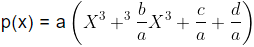Where,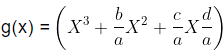i.e., first make the coefficient of x= equal to 1 if it is not 1, then find the constant term.
Step II: Find all the possible factors of constant term (d / a ) of g(X).
Step III: Check at which factor of constant term, p(x) is zero and get one factor of p(x), (i.e., x − α)
Step IV: Write p(x) as the product of this factor (x − α) and a quadratic polynomial i.e., p(x) = (x − α) (a1x2 + b1x + c1)
Step V: Apply splitting method of middle term or factor theorem in quadratic polynomial to get the other two factors. Thus, we get all the three factors of given the cubic polynomial.

Using factor theorem, factorise x3 + 13x2 + 32x + 20

Let p(x) = x3 + 13x2 + 32x + 20
Here the constant term = 20 and the coefficient of x3 is 1.
All possible factors of 20 are ±1, ±2, ±4, ±5, ±10, and ± 20
At x = −1,
p(x) = x3 + 13x2 + 32x + 20
p(−1) = (−1)3 + 13(−1)2 + 32(−1) + 20
= −1 + 13 − 32 + 20
= 33 − 33
= 0
So, we find p(−1) = 0 So, (x + 1) is a factor of p(x)

Now, x2 + 12x + 20 can be factorised by splitting the middle term,
we get,
x+ 12x + 20
= x2 + 10x + 2x + 20
= x(x + 10) + 2(x + 10)
= (x + 2)(x + 10) ...........(ii)
From equation (i) and (ii) we get,
x+ 13x2 + 32x + 20 = (x + 1)(x + 2)(x + 10)
If p(y) = y3 −4y2 + y + 6 then show that p(3) = 0 and hence factorise p(y).
Given: p(y) = y3 − 4y2 + y + 6...... (i)
Put y = 3 in equation (i), we get
p(3) = (3)3 − 4(3)2 + 3 + 6
= 27 − 36 + 3 + 6 = 0
Since, p(3) = 0, therefore y – 3 is a factor of p(y).
∴ p(y) = (y − 3)(y2 − y − 2)
∴ p(y) = (y − 3)(y2 − 2y + y − 2)
[∵ −2 + 1 = −1 and − 2 × 1 = −2]
= (y − 3)[y(y − 2) + 1(y − 2])
= (y − 3)(y + 1)(y − 2)

Question for Chapter Notes: Polynomials
Try yourself:Which of the following methods can be used for the factorization of a polynomial?

### Algebraic Identities

An algebraic identity is an equality which is true for all values of the variables occurring in it. Some algebraic identities are given below: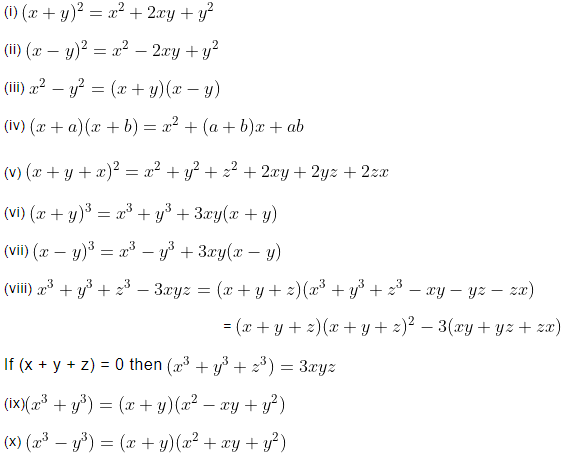Problem based on Algebraic Identities
Example:
(i) (x + 4)2
(ii) (x − 2)2

(i) (x + 4)

= x2 + 2 × x × 4 + 42 [Using identity (x + y)2  = x2 + 2xy + y2  ]
= x2 + 8x + 16

(ii)(x − 2)2

= x2 + 2 × x × 2 + 22 [Using identity (x + y)2  = x2 + 2xy + y2 ]
= x+ 4x + 4

Example: Find the value of the polynomial X2 - 16, for x  =96

Putting x = 96  in the given polynomial we get,
x2 − 16 = (96)2 − (4)2
[Using identity x− y2 = (x + y)(x − y)]
= (96 + 4)(96 − 4)
= 100 × 92
= 9200

Evaluate by using identity 149 x 151

149 × 151 = (150 − 1)(150 + 1)
= (150)− (1)2
= 22500 − 1
= 22499

Expand using suitable identity (2x - y + z)2

(2x – y + z)2 = [2x + (−y) + z]2
[Using identity: (x + y + z)2 = x2 + y2 + z2 + 2xy + 2yz+ 2zx]
= (2x)2 + (−y)2 + z2 + 2 × 2x × (−y) + 2 × (−y) × z + 2 × 2x × z
= 4x( + y2 + z2 − 4xy − 2yz + 4xz

Expand using suitable identity (102)3

(102)3 = (100 + 2)3 [Using identity (x + y)3 = x3 + y3 + 3xy(x + y)]
= 1003 + 23 + 3 × 100 × 2(100 + 2)
= 1000000 + 8 + 600 × 102
= 1000000 + 8 + 61200
= 1061208

If x = 2 + y, then what is the value of x3 − y3 − 6xy

x = 3 + y
x – y = 2
(x − y)3 = (2)3
x3 − y3 − 3xy × 2 = 8
x3 − y3− 6xy = 8

If a2 + b2 + c2 = 160 and ab + bc + ca = 9/2  then find the value of (a + b + c)3

(a + b + c)2 = a2 + b+ c2 + 2(ab + bc + ca)
= 160 + 2 ×9/2
(a + b + c)2 = 160 + 9
(a + b + c)2 = 169
a + b + c = √169
a + b + c = 13
Hence, (a + b + c)= (13)3= 2197

### Remainder Theorem (Old Syllabus)

Before we start remainder theorem we need to know about factors and multiples, long division algorithm.

Factors and Multiples
If a number is divided by another number exactly, without leaving any non-zero remainder, then the number which divides is called a factor of the number and the number that has been divided is known as the multiple of the number.
Example:

Suppose we divide 3y3 + y2 +y by y, we get,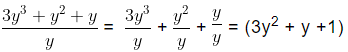Here, remainder is zero, so y divides (3y3 + y2 +y) exactly. Thus, y is a factor of (3y3 + y2 +y)  and (3y+ y2 +y) is a multiple of y.  So, (3y2 + y +1) is also a factor of (3y3 + y2 +y). Hence, y and (3y2 + y +1) are factors of (3y+ y+y). (3y3 + y2 +y) is a multiple of y as well as  (3y2 + y +1)

Long Division Algorithm
If p(x) and g(x) are any two polynomials with g(x) ≠ 0, then we can find the polynomial q(x) and r(x) such that,
p(x) = g(x) × q(x) + r(x)
p(x) → Dividend
g(x) → Divisor
q(x) → Quotient
r(x) → Remainder

Dividend = Divisor × Quotient + Remainder

• Here, p(x) when divided by g(x) gives q(x) as quotient and r(x) as remainder.
• Where r(x) = 0 or degree of r(x) < degree of g(x) This result is known as the Long Division Algorithm First we will study the method of dividing one polynomial by another polynomial with the help of an example.

Divide p(x) by g(x), where p(x) = 3x + 4x2+ 1 and g(x) = −1+ x

• Firstly, arrange the terms of the dividend and the divisor in descending order of the degrees (also known as writing the polynomial in standard form). We get,
p(x) = 4x2 + 3x + 1 and g(x) = x − 1
• To obtain the first term of the quotient, we will divide the highest degree term of the dividend, x& by the highest degree term of divisor, x. We get the first term of the quotient, 4x and carry out the division process, what remain is (7x + 1).

The first term of the dividend is 4xand the first term of the divisor is x, since, 4x2 / x = 4 (first term of quotient)
Multiply the divisor (x − 1) by the first term 4x of the quotient and then subtract from dividend.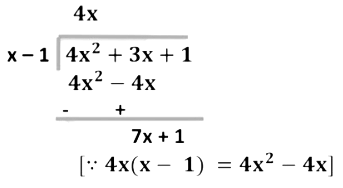• To obtain the second term of the quotient divide the highest degree term of the new dividend 7x by the highest degree term of the divisor x. We get −7x and what remain is 8. As the degree of 8 is equal to the degree of the divisor,(x − 1) we will continue division process.
The first term of the new dividend is 7x and the first term of the divisor is x, since, 7X/X = 7 = second term of quotient.
Multiplying the divisor (x − 1) by the second term of quotient, 7 and then subtracting from the new dividend, we get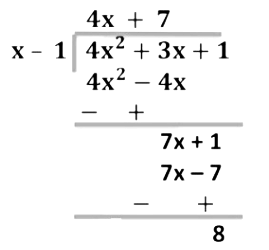• The remainder is 8. As the degree of remainder is less than the divisor, we stop our division process.
Dividend p(x) = 4x2+ 3x + 1
Divisor g(x) = x + 1
Quotient q(x) = 4x + 7
Remainder r(x) = 8
p(x) = g(x) × q(x) + r(x)
Thus, 4x2 + 3x + 1 = (x - 1)(4x + 7) + 8
i.e., Dividend = Divisor × Quotient + Remainder

Find the remainder and quotient when p(t) = t+ t+ 2t + 3  is divided by t + 2 by actual division method.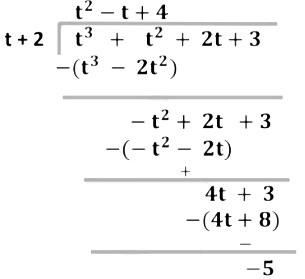Here, the remainder is – 5 and quotient is t& − t + 4

Remainder Theorem
Let p(x) be a polynomial of degree n greater than or equal to 1 (i.e., n ≥ 0) and a be any real number.
If p(x) is divided by the linear polynomial (x − a), then the remainder is p(a)

Proof:

Let p(x) be any polynomial with degree greater than or equal to 1.
We suppose that when p(x) is divided by x – a, the quotient is
q(x) and the remainder is r(x),
i. e. , p(x) = [(x – a) q(x)] + r(x) ... ... . (1)
The degree of (x – a) = 1 and the degree of r(x) is 0.
We see that the degree of r(x) is less than the degree of x – a.
This means that r(x) is a constant, equal to r.
For every value of x, r(x) = r,
On putting r(x) = r in equation (1), we get
p(x) = [(x – a) q(x)] + r) ... ... . (2)
If x = a, then equation (2) becomes
p(a) = (a – a) q(a) + r
p(a) = r

Example: Find the remainder when the polynomial p(x) = x4 − 3x2 + 2x + 1 is divided by x - 2

By remainder theorem, if p(x) is divided by the linear polynomial (x − a), then the remainder is p(a). Therefore, the remainder of the polynomial, p(x) = x4 − 3x2 + 2x + 1 is p(2).
p(x) = x4 − 3x2 + 2x + 1
p(2) = (2)4 − 3(2)2 + 2(2) + 1
p(1) = 16 − 12 + 4 + 1 = 9
The required remainder, p(2) = 9

Example: Find the remainder when the polynomial p(x) = 4x3 − 12x2 + 14x − 3 is divided by g(x) = x − {1/2}

By remainder theorem,
We know that when the polynomial, p(x) is divided by g(x) = ( X -1/2 ) then the remainder is equal to p (1/2)
p(x) = 4x3 − 12x2 + 14x − 3

p(1/2) = 4(1/2)3- 12(1/2)2+14(1/2) -3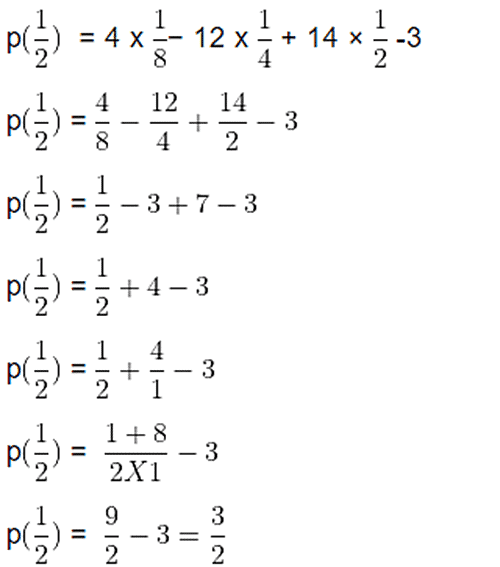Hence, required remainder, p (1/2) =3/2

Example: Find the value of k, if X21 + 2x20 +3x + k is divisible by x + 1.

Let p(x) =  X21 + 2x20 +3x + k
Given: p(x) is divisible by x + 1
Here,
The polynomial  X21 + 2x20 +3x + k  is divided by x + 1.Therefore the remainder is equal to −1.
Putting x = −1 in the given polynomial, we get
p(−1) = 0
p(−1) = (-1)21+ 2(-1)20+3(-1)+k
p(−1) =−1 + 2 − 3 + k
p(−1) = −4 + 2 + k
p(−1) = −2 + k
p(−1) = k − 2
We know that p(−1) = 0
k − 2 = 0
k = 2

Example: Check whether 2t + 1 is a factor of 4t2 − 2t  or not.

Given polynomial is q(t) = 4t2 − 2t ....(i)
Let, g(t) = 2t + 1
To find the zero of q(t), we put g(t) = 0
∴ 2t + 1 = 0 → t = −1/2
On putting, t = − 1/2 in equation (i) we get,
q (-1/2) = 4 (-1/2})2 - 2 (1/2)
= 4 x 1/4 - 2  (1/2)
=1 - 1
= 0
At t = -1/2  , we get q (-1/2}) = 0
i.e., remainder on dividing q(t) by g(t) is 0.
Hence, (2t + 1) is a factor of q(t) i. e. , q(t) is a multiple of (2t + 1)

Question for Chapter Notes: Polynomials
Try yourself:If f(x) is a polynomial and g(x) is a non-zero polynomial with the same degree as f(x), then the remainder when f(x) is divided by g(x) is:

The document Polynomials Chapter Notes | Mathematics (Maths) Class 9 is a part of the Class 9 Course Mathematics (Maths) Class 9.
All you need of Class 9 at this link: Class 9

## FAQs on Polynomials Chapter Notes - Mathematics (Maths) Class 9

 1. What is a polynomial?Ans. A polynomial is an algebraic expression consisting of variables, coefficients, and exponents, combined using addition, subtraction, multiplication, and non-negative integer exponents. It can have one or more terms, and the degree of a polynomial is determined by the highest exponent of the variable.
 2. How can we identify a polynomial?Ans. To identify a polynomial, we check if the expression satisfies the following conditions: it must have only non-negative integer exponents, it must have finite terms, and all the variables must be raised to non-negative integer exponents. If these conditions are met, the expression can be considered a polynomial.
 3. What is the degree of a polynomial?Ans. The degree of a polynomial is the highest exponent of the variable in the expression. It indicates the highest power to which the variable is raised. For example, if the highest exponent is 3, then the polynomial has a degree of 3.
 4. How can we add or subtract polynomials?Ans. To add or subtract polynomials, we combine like terms. Like terms are those that have the same variable(s) raised to the same exponent(s). We simply add or subtract the coefficients of these like terms while keeping the variables and exponents unchanged.
 5. What are the different types of polynomials?Ans. Polynomials can be classified based on their number of terms. Some common types of polynomials include: - Monomial: A polynomial with only one term. - Binomial: A polynomial with two terms. - Trinomial: A polynomial with three terms. - Quadratic polynomial: A polynomial of degree 2. - Cubic polynomial: A polynomial of degree 3. - Linear polynomial: A polynomial of degree 1. - Constant polynomial: A polynomial with no variable terms, only a constant term.

## Mathematics (Maths) Class 9

62 videos|426 docs|102 tests

## Mathematics (Maths) Class 9

62 videos|426 docs|102 tests
Signup to see your scores go up within 7 days! Learn & Practice with 1000+ FREE Notes, Videos & Tests.
10M+ students study on EduRev
Track your progress, build streaks, highlight & save important lessons and more!(Scan QR code)
Related Searches

,

,

,

,

,

,

,

,

,

,

,

,

,

,

,

,

,

,

,

,

,

;